### Moment of Inertia, Section Modulus, Radii of Gyration Equations Square and Rectagular Sections

Strength / Mechanics of Materials Table of Content

Moment of Inertia, Section Modulus, Radii of Gyration Equations and calculators or Square and Rectagular Sections .

 A = Area (in2, mm2) I = Moment of Inertia (in4, mm4) Gr = Radius of Gyration = (in, mm) y = Distance of Axis to Extreme Fiber (in, mm)

 Section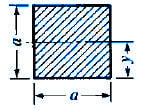Open Section Properties Case 1 Calculator Moment of InertiaRadius of GyrationAreaySectionOpen Section Properties Case 2 Calculator Moment of InertiaRadius of GyrationAreaySection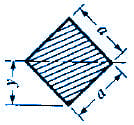Open Section Properties Case 3 Calculator Moment of InertiaRadius of GyrationAreaySectionOpen Section Properties Case 4 Calculator Moment of InertiaRadius of GyrationAreaySection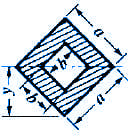Open Section Properties Case 5 Calculator Moment of InertiaRadius of GyrationAreaySection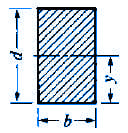Open Section Properties Case 6 Calculator Moment of InertiaRadius of GyrationAreaySection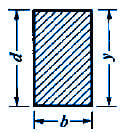Open Section Properties Case 7 Calculator Moment of InertiaRadius of GyrationAreaySectionOpen Section Properties Case 8 Calculator Moment of InertiaRadius of GyrationAreaySection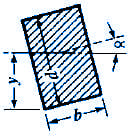Open Section Properties Case 9 Calculator Moment of InertiaRadius of GyrationAreaySection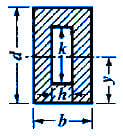Open Section Properties Case 10 Calculator Moment of InertiaRadius of GyrationAreayRelated: